# ML | Principal Component Analysis(PCA)

Principal Component Analysis (PCA) is a statistical procedure that uses an orthogonal transformation which converts a set of correlated variables to a set of uncorrelated variables. PCA is a most widely used tool in exploratory data analysis and in machine learning for predictive models. Moreover, PCA is an unsupervised statistical technique used to examine the interrelations among a set of variables. It is also known as a general factor analysis where regression determines a line of best fit.

Module Needed:

 `import` `pandas as pd ` `import` `numpy as np ` `import` `matplotlib.pyplot as plt ` `import` `seaborn as sns ` `%``matplotlib inline `

Code #1:

 `# Here we are using inbuilt dataset of scikit learn ` `from` `sklearn.datasets ``import` `load_breast_cancer ` ` `  `# instantiating ` `cancer ``=` `load_breast_cancer() ` ` `  `# creating dataframe ` `df ``=` `pd.DataFrame(cancer[``'data'``], columns ``=` `cancer[``'feature_names'``]) ` ` `  `# checking head of dataframe ` `df.head() `

Output: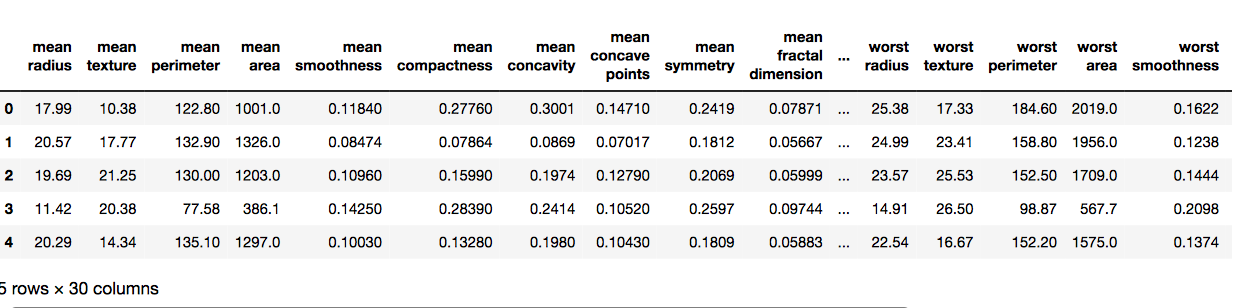Code #2:

 `# Importing standardscalar module  ` `from` `sklearn.preprocessing ``import` `StandardScaler ` ` `  `scalar ``=` `StandardScaler() ` ` `  `# fitting ` `scalar.fit(df) ` `scaled_data ``=` `scalar.transform(df) ` ` `  `# Importing PCA ` `from` `sklearn.decomposition ``import` `PCA ` ` `  `# Let's say, components = 2 ` `pca ``=` `PCA(n_components ``=` `2``) ` `pca.fit(scaled_data) ` `x_pca ``=` `pca.transform(scaled_data) ` ` `  `x_pca.shape `

Output:
# Reduced to 569, 2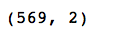`# giving a larger plot ` `plt.figure(figsize ``=``(``8``, ``6``)) ` ` `  `plt.scatter(x_pca[:, ``0``], x_pca[:, ``1``], c ``=` `cancer[``'target'``], cmap ``=``'plasma'``) ` ` `  `# labeling x and y axes ` `plt.xlabel(``'First Principal Component'``) ` `plt.ylabel(``'Second Principal Component'``) `

Output: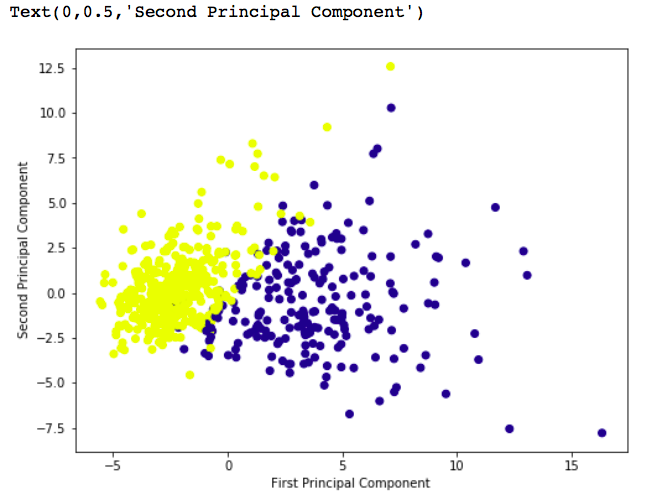`# components ` `pca.components_ `

Output: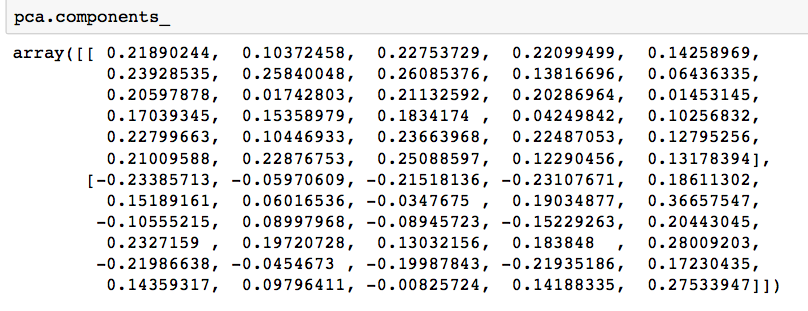`df_comp ``=` `pd.DataFrame(pca.components_, columns ``=` `cancer[``'feature_names'``]) ` ` `  `plt.figure(figsize ``=``(``14``, ``6``)) ` ` `  `# plotting heatmap ` `sns.heatmap(df_comp) `

Output: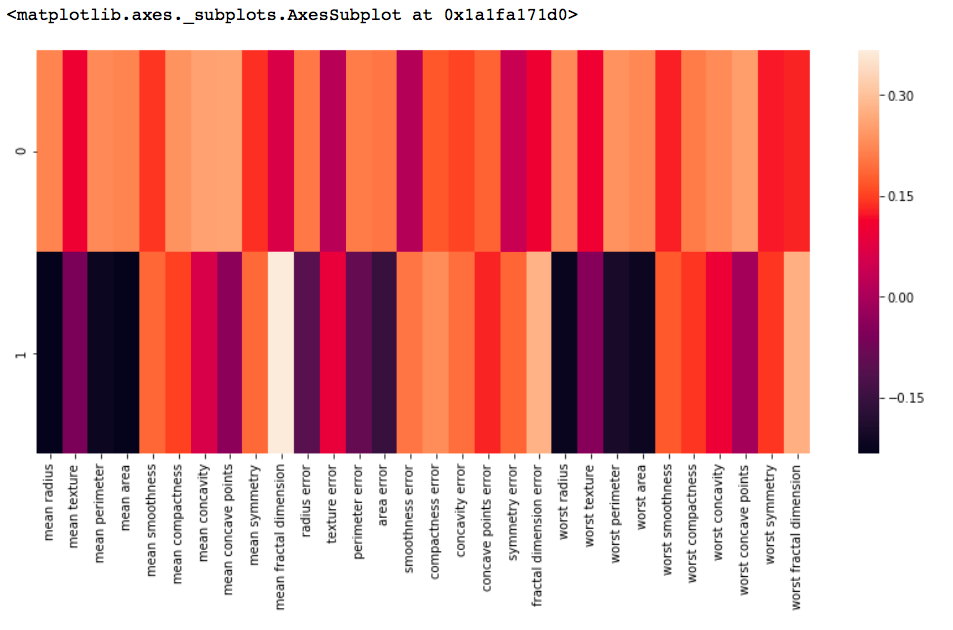My Personal Notes arrow_drop_upCheck out this Author's contributed articles.

If you like GeeksforGeeks and would like to contribute, you can also write an article using contribute.geeksforgeeks.org or mail your article to contribute@geeksforgeeks.org. See your article appearing on the GeeksforGeeks main page and help other Geeks.

Please Improve this article if you find anything incorrect by clicking on the "Improve Article" button below.